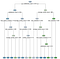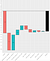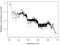# NEW R package that makes XGBoost interpretable

## xgboostExplainer makes your XGBoost model as transparent and 'white-box' as a single decision tree

In this post I'm going to do three things:

1. Show you how a single decision tree is not great at predicting, but it is very interpretable
2. Show you how xgboost (an ensemble of decision trees) is very good at predicting, but not very interpretable
3. Show you how to make your xgboost model as interpretable as a single decision tree, by using the new xgboostExplainer package

Code and links to the package are included at the bottom of this post.

For the demonstration, I'll use a dataset from Kaggle, to predict employee attrition from a fictional company.

First up, the decision tree!

# Decision Tree (AUC: 0.823)A decision tree to predict employee attrition. The prediction is the label on each leaf node (eg 0.59 means 59% chance of leaving)

Predictions made using this tree are entirely transparent - ie you can say exactly how each feature has influenced the prediction.

Let's say we have an employee with the following attributes:

`Satisfaction Level = 0.23 Average Monthly Hours = 200 Last Evaluation = 0.5`

The model would estimate the likelihood of this employee leaving at 0.31 (ie 31%). By following this employee's path through the tree, we can easily see the contribution of each feature.

`0.24 ::: Baseline +0.28 ::: Satisfaction Level (prediction is now 0.52) -0.09 ::: Average Monthly Hours (prediction is now 0.43) -0.12 ::: Last Evaluation (prediction is now 0.31)= 0.31 ::: Prediction`

Therefore the model would say that the poor satisfaction score is making it more likely that the employee is going to leave (+0.28), whereas the average monthly and last evaluation scores actually decrease the likelihood of leaving (by -0.09 and -0.12 respectively). )

It is easy to see why a decision tree model is appealing to decision makers in a business. You can pull apart the model and see what variables are influencing the prediction for every single employee, making direct action possible.

The problem is that decision trees are generally not that great in terms of predictive power.

The decision tree scores 0.823 AUC, which is ok, but could be better - any further growth of the tree would have led to overfitting.

Let's see what xgboost makes of it ...

# XGBoost (AUC: 0.919)

So how interpretable is this model? Can we break apart each prediction like we could with a decision tree? The short answer is no, there's no functionality for this out of the box, either R or Python.

To see what this means, let's take this employee:

When you push this through the xgboost model, you get a 21.4% likelihood of leaving.

However, since there are now 42 trees, each contributing to the prediction, it becomes more difficult to judge the influence of each individual feature.

So if your head of HR wanted to know why this employee had a 21.4% chance of leaving, even though their satisfaction score is 0.639, which is quite high, you'd have to shrug your shoulders and say that the model is' black box 'and not interpretable.

The best you could do is show this 'importance' graph of each variable, which is built into the xgboost framework.

This tells you that satisfaction level is the most important variable across all predictions, but there's no guarantee it's the most important for this particular employee. Also, good luck trying to explain what the x-axis means to your senior stakeholder. It is the Gain contribution of each feature to the model, where Gain is defined as:

So probably a non-starter.

# Now what?

The question is, can we make XGBoost as transparent as the single decision tree?

# The XGBoost Explainer

Let's first see it in action.

Take the last employee with the 21.4% likelihood of leaving - through the lens of the XGBoost Explainer, this is what you get back:The explanation of the log-odds prediction of -1.299 (y-axis shows the probability, the bar labels show the log-odds impact of each variable)

The prediction of 21.4% is broken down into the impact of each individual feature. More specifically, it breaks down the log-odds of the prediction, which in this case is -1.299.

Walking through step by step, just like we did for the decision tree, except this time working with log-odds:

`-1.41 ::: Baseline (Intercept) -1.10 ::: Satisfaction Level (prediction is now -2.51) +0.98 ::: Last Evaluation (prediction is now -1.53) +0.32 ::: Time Spent At Company (prediction is now - 1.21) +0.27 ::: Hours Average Monthly (prediction is now -0.94) -0.24 ::: Sales (prediction is now -1.18) -0.18 ::: Number of Projects (prediction is now -1.36)+0.11 ::: Work Accident (prediction is now -1.25) -0.07 ::: Salary (prediction is now -1.32) +0.02 ::: Promotion Last 5 Years (prediction is now -1.30)= -1.299 ::: Prediction`

The probability is then just the logistic function applied to the log-odds.

The waterfall chart above shows how probability of leaving changes with the addition of each variable, in reverse order of the absolute impact on the log-odds. The intercept reflects the fact that this is an imbalanced dataset - only around 20% of employees in the training set were leavers.

It’s now clear that the high satisfaction score of the employee does indeed pull the predicted log-odds down (by-1.1), but this is more than cancelled out by the impacts of the last evaluation, time at company and average hours variables, all of which pull the log-odds up.

Let's take a moment to reflect on what this means. It means you can build powerful models using xgboost and fully understand how every single prediction is made. Hopefully you're as excited about this as I am!

# How does it work?

The R xgboost package contains a function 'xgb.model.dt.tree' that exposes the calculations that the algorithm is using to generate predictions. The xgboostExplainer package extends this, performing the additional calculations necessary to express each prediction as the sum of feature impacts.

It is worth noting that the 'impacts' here are not static coefficients as in a logistic regression. The impact of a feature is dependent on the specific path that the observation took through the ensemble of trees.

In fact, we can plot the impact against the value of the variable, for say, Satisfaction Level and get insightful graphs like this:Each point is one employee from the test set. The satisfaction level of the employee is plotted on the x-axis; the impact of the satisfaction level on the log-odds of leaving is plotted on the y-axis.

Things to note:

1. If this were a logistic regression, the points would be on a straight line, with the slope dependent on the value of the coefficient
2. If this were a decision tree, the points would lie on a set of horizontal lines (see plot below, in red)
3. The xgboostExplainer beautifully captures the non-linearity, but is not restricted by the requirement of a single straight line or steps.

Let's try another variable - last evaluation:

On first inspection, this doesn’t look too interesting. It basically says that there is large variance in the impact of the last evaluation variable, except perhaps for values ​​around 0.5, where the impact is more likely to be close to zero.

However, if we colour the plot by satisfaction level <0.5 (blue) or ≥0.5 (red), the story becomes very clear ...

Employees that are happy (red) are more likely to leave after a higher last evaluation score (i.e. talented happy employees are more likely to be poached than less talented happy employees). However, employees that are unhappy (blue), are more likely to leave after a lower last evaluation score (i.e. the poor evaluation score may be due to a lack of motivation, resulting in the employee switching jobs).

Perhaps worryingly for this fictional company, the model is judging that employees who are happy but scored poorly on the last evaluation are more likely to stick around and not leave.

The two diagonally crossing clouds of red and blue points make this evident.

What's incredible is that we can draw this insight directly from the xgboost model, using the xgboostExplainer.

In other words, your exploratory analysis no longer needs to be separated from your predictive modelling. By using the xgboostExplainer package, the model itself may start to tell you things about your data that you didn’t know, leading to a much deeper understanding of the problem.

# How can I get it?

You can install it by using the devtools package in R:

`install.packages("devtools") library(devtools) install_github("AppliedDataSciencePartners/xgboostExplainer")`

Check out the docs for how to build an xgboostExplainer on your own xgboost models.

# Code for the above examples

The R code for the employee attrition analysis is:

`library(data.table)library(rpart)library(rpart.plot)library(caret)library(xgboost)library(pROC)set.seed(123)full = fread('./data/HR.csv', stringsAsFactors = T)full = full[sample(.N)]#### Add Random Noisetmp_std = sd(full[,satisfaction_level])full[,satisfaction_level:=satisfaction_level + runif(.N,-tmp_std,tmp_std)]full[,satisfaction_level:=satisfaction_level - min(satisfaction_level)]full[,satisfaction_level:=satisfaction_level / max(satisfaction_level)]tmp_std = sd(full[,last_evaluation])full[,last_evaluation:=last_evaluation + runif(.N,-tmp_std,tmp_std) ]full[,last_evaluation:=last_evaluation - min(last_evaluation)]full[,last_evaluation:=last_evaluation / max(last_evaluation)]tmp_min = min(full[,number_project])tmp_std = sd(full[,number_project])full[,number_project:=number_project + sample(-ceiling(tmp_std):ceiling(tmp_std),.N, replace=T)]full[,number_project:=number_project - min(number_project) + tmp_min]tmp_min = min(full[,average_montly_hours])tmp_std = sd(full[,average_montly_hours])full[,average_montly_hours:=average_montly_hours + sample(-ceiling(tmp_std):ceiling(tmp_std),.N, replace=T)]full[,average_montly_hours:=average_montly_hours - min(average_montly_hours) + tmp_min]tmp_min = min(full[,time_spend_company])tmp_std = sd(full[,time_spend_company])full[,time_spend_company:=time_spend_company + sample(-ceiling(tmp_std):ceiling(tmp_std),.N, replace=T)]full[,time_spend_company:=time_spend_company - min(time_spend_company) + tmp_min]tmp_min = min(full[,number_project])tmp_std = sd(full[,number_project])full[,number_project:=number_project + sample(-ceiling(tmp_std):ceiling(tmp_std),.N, replace=T)]full[,number_project:=number_project - min(number_project) + tmp_min]#### Create Train / Test and Foldstrain = full[1:12000]test = full[12001:14999]cv <- createFolds(train[,left], k = 10)# Controlctrl <- trainControl(method = "cv",index = cv)#### Train Treetree.cv <- train(x = train[,-"left"], y = as.factor(train[,left]), method = "rpart2", tuneLength = 7,                 trControl = ctrl, control = rpart.control())tree.model = tree.cv\$finalModelrpart.plot(tree.model, type = 2,extra =  7,fallen.leaves = T)rpart.plot(tree.model, type = 2,extra =  2,fallen.leaves = T)tree.preds = predict(tree.model, test)[,2]tree.roc_obj <- roc(test[,left], tree.preds)cat("Tree AUC ", auc(tree.roc_obj))#### Train XGBoostxgb.train.data = xgb.DMatrix(data.matrix(train[,-'left']), label = train[,left], missing = NA)param <- list(objective = "binary:logistic", base_score = 0.5)xgboost.cv = xgb.cv(param=param, data = xgb.train.data, folds = cv, nrounds = 1500, early_stopping_rounds = 100, metrics='auc')best_iteration = xgboost.cv\$best_iterationxgb.model <- xgboost(param =param,  data = xgb.train.data, nrounds=best_iteration)xgb.test.data = xgb.DMatrix(data.matrix(test[,-'left']), missing = NA)xgb.preds = predict(xgb.model, xgb.test.data)xgb.roc_obj <- roc(test[,left], xgb.preds)cat("Tree AUC ", auc(tree.roc_obj))cat("XGB AUC ", auc(xgb.roc_obj))#### Xgb importancecol_names = attr(xgb.train.data, ".Dimnames")[]imp = xgb.importance(col_names, xgb.model)xgb.plot.importance(imp)#### THE XGBoost Explainerlibrary(xgboostExplainer)explainer = buildExplainer(xgb.model,xgb.train.data, type="binary", base_score = 0.5, trees_idx = NULL)pred.breakdown = explainPredictions(xgb.model, explainer, xgb.test.data)cat('Breakdown Complete','\n')weights = rowSums(pred.breakdown)pred.xgb = 1/(1+exp(-weights))cat(max(xgb.preds-pred.xgb),'\n')idx_to_get = as.integer(802)test[idx_to_get,-"left"]showWaterfall(xgb.model, explainer, xgb.test.data, data.matrix(test[,-'left']) ,idx_to_get, type = "binary")####### IMPACT AGAINST VARIABLE VALUEplot(test[,satisfaction_level], pred.breakdown[,satisfaction_level], cex=0.4, pch=16, xlab = "Satisfaction Level", ylab = "Satisfaction Level impact on log-odds")plot(test[,last_evaluation], pred.breakdown[,last_evaluation], cex=0.4, pch=16, xlab = "Last evaluation", ylab = "Last evaluation impact on log-odds")cr <- colorRamp(c("blue", "red"))plot(test[,last_evaluation], pred.breakdown[,last_evaluation], col = rgb(cr(round(test[,satisfaction_level])), max=255), cex=0.4, pch=16, xlab = "Last evaluation", ylab = "Last evaluation impact on log-odds")`

Hopefully you will find the xgboostExplainer useful - I would love to hear from you if you would like to contribute to the project or if you have any suggestions.

If you would like to learn more about how our company, Applied Data Science develops innovative data science solutions for businesses, feel free to get in touch through our website or directly through LinkedIn.

... and if you like this, feel free to leave a few hearty claps :)

Applied Data Science is a London based consultancy that implements end-to-end data science solutions for businesses, delivering measurable value. If you’re looking to do more with your data, let's talk.

Written by

Written by

## David Foster

#### Founding Partner of Applied Data Science Partners